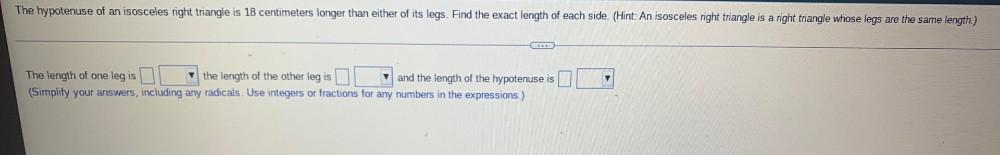Question:

# The hypotenuse of an isosceles right triangle is 18 centimeters longer than either of its legs. Find the exact length of each side. (Hint: An isosceles right triangle is a right triangle whose legs arThe hypotenuse of an isosceles right triangle is 18 centimeters longer than either of its legs. Find the exact length of each side. (Hint: An isosceles right triangle is a right triangle whose legs are the same length.) The length of one leg is____ the length of the other leg is______ and the length of the hypotenuse is______